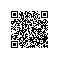# 路由设计

Route::post('/admin/validate', ['uses' => 'AdminController@postValidate', 'permissions'=>['admin.validate', 'admin.index']]);

# 中间件设计

<?php namespace App\Http\Middleware;

use Closure;

class Permission {

/**
* Handle an incoming request.
*
* @param  \Illuminate\Http\Request  $request * @param \Closure$next
* @return mixed
*/
public function handle($request, Closure$next)
{
$permits =$this->getPermission($request);$admin = \App\Http\Middleware\Authenticate::getAuthUser();

// 只要有一个有权限，就可以进入这个请求
foreach ($permits as$permit) {
if ($permit == '*') { return$next($request); } if ($admin->hasPermission($permit)) { return$next($request); } } echo "没有权限，请联系管理员";exit; } // 获取当前路由需要的权限 public function getPermission($request)
{
$actions =$request->route()->getAction();
if (empty($actions['permissions'])) { echo "路由没有设置权限";exit; } return$actions['permissions'];
}
}

// 判断是否有某个权限
public function hasPermission($permission) {$permission_db = $this->permissions; if(in_array($permission, $permission_db)) { return true; } return false; } // permission 是一个二维数组 public function getPermissionsAttribute($value)
{
if (empty($value)) { return []; }$data = json_decode($value, true);$ret = [];
foreach ($data as$key => $value) {$ret[] = $key; foreach ($value as $value2) {$ret[] = "{$key}.{$value2}";
}
}
return array_unique($ret); } // 全局设置permission public function setPermissionsAttribute($value)
{
$ret = []; foreach ($value as $item) {$keys = explode('.', $item); if (count($keys) != 2) {
continue;
}
$ret[$keys][] = $keys; }$this->attributes['permissions'] = json_encode($ret); } } 在数据库中，我将二维数组存储为json，利用laravel的Attribute的get和set方法，完成了数据库中json和外界程序逻辑的连接。然后hasPermission就显得很轻松了，直接判断in_array就ok了。 # 后续 这个权限认证的逻辑就清晰了。然后如果页面中某个tab或者nav需要对不同权限的用户展示，只需要在view中判断 @if ($admin->hasPermission('admin.index'))
@endif

# 总结使用钉钉扫一扫加入圈子
+ 订阅